Problem: Decide whether the following integral converges: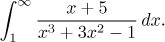Solution: There is just one problem, the infinity. So this is an improper integral of the basic type with a positive function, and we can use convergence tests. When x grows to infinity, in the numerator and the denominator the highest powers prevail, after cancelling we can guess that our function looks about the same as 1/x2 around infinity. Since we know that the latter function has a convergent integral to infinity, we guess that also the given integral converges.

If we want to prove this fact using the Comparison test, we would need to find an upper estimate. Unfortunately, this is not all that simple, since the desired inequality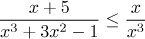is definitely not true (check). So instead we try the Limit Comparison test with our guess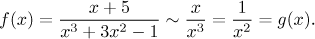This guess has to be justified: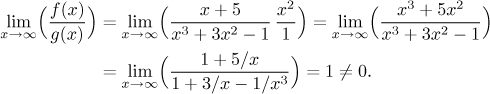The limit condition is satisfied. Since we know that the test integral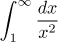converges, by the Limit Comparison test, the given integral also converges.